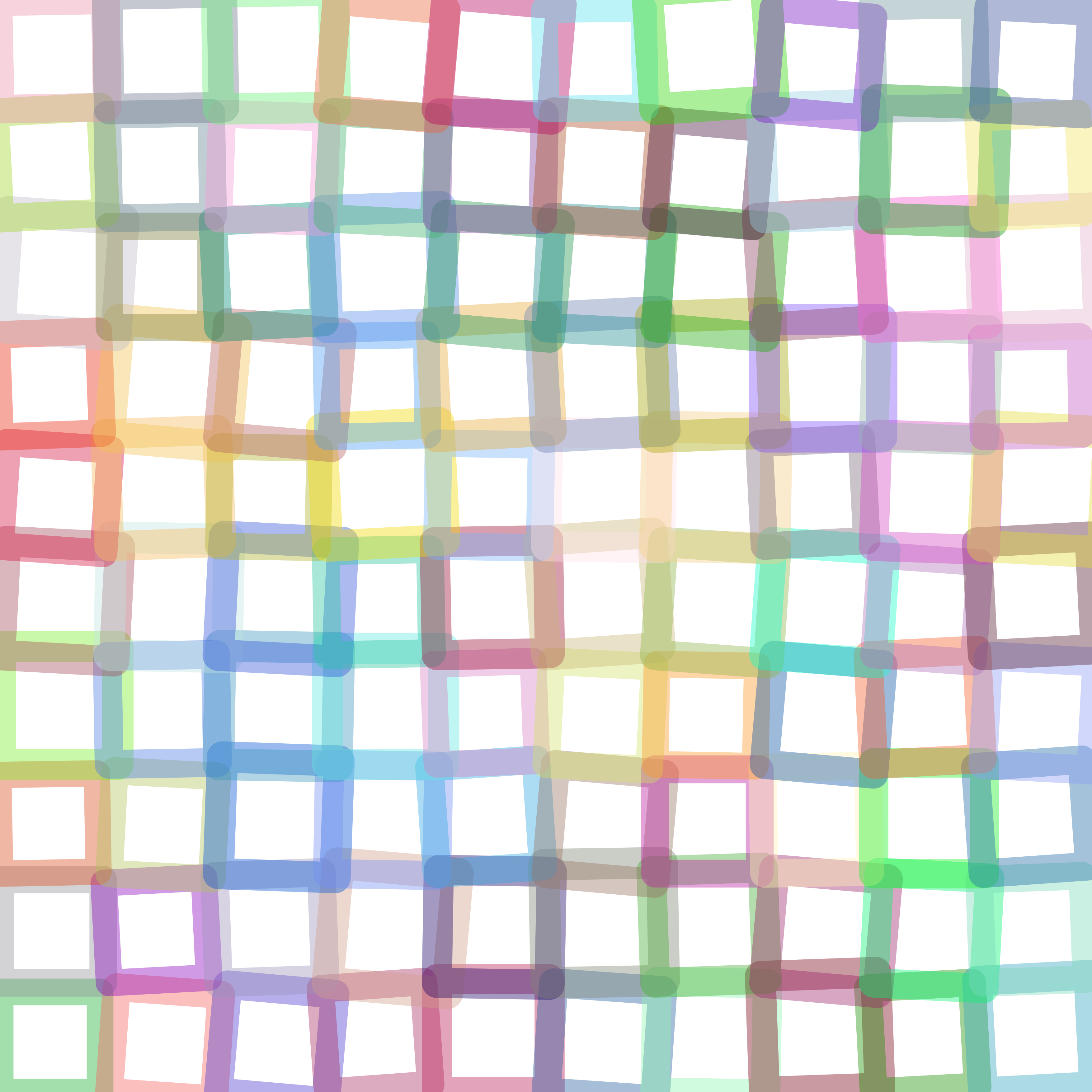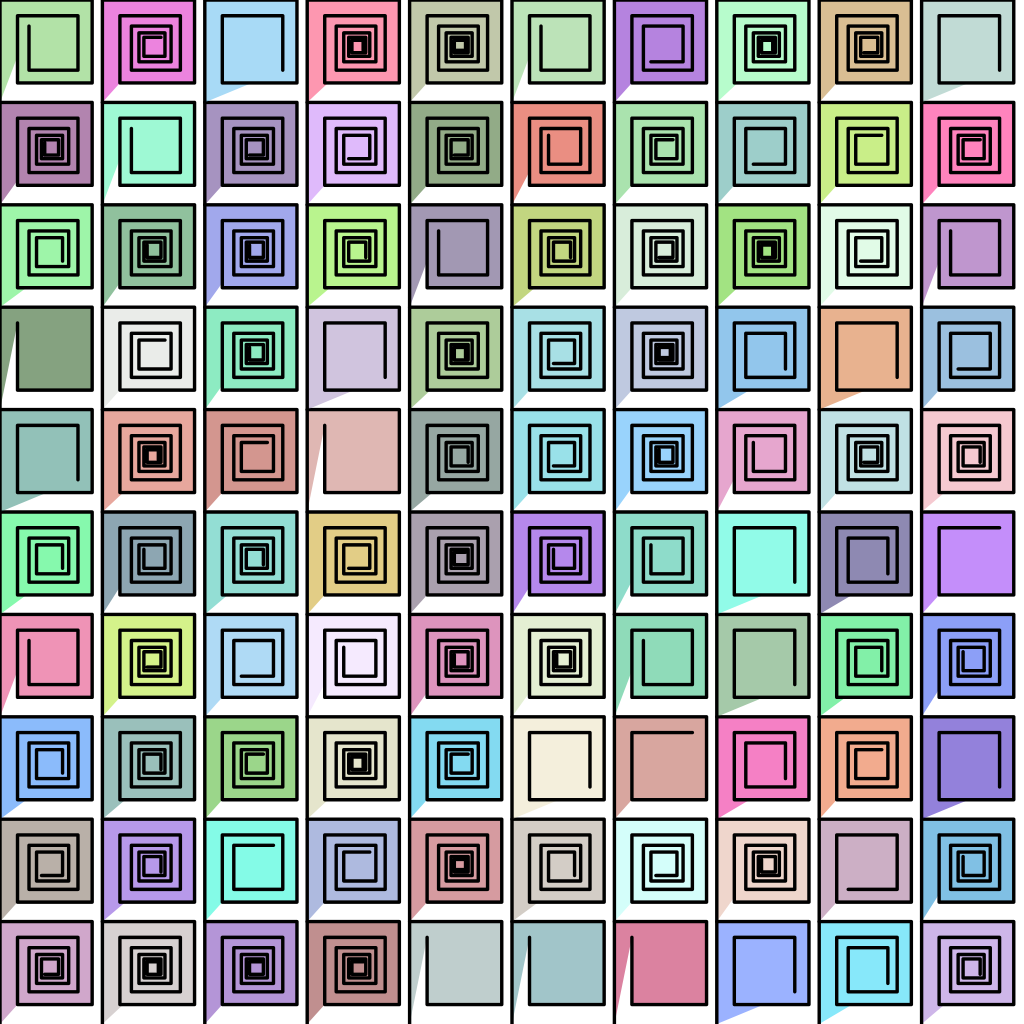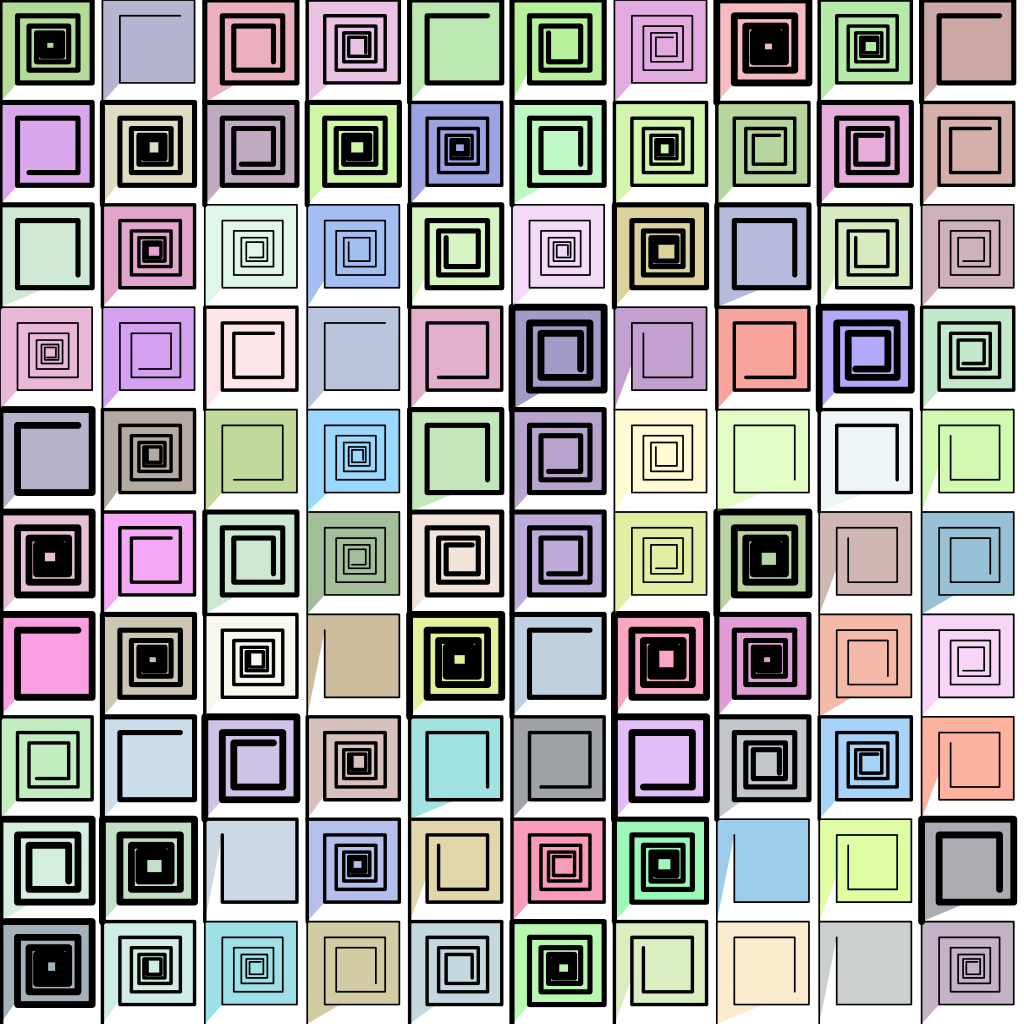## Rectangular grids

This activity has the following desired goals:

• Learning to draw a rectangular grid using the turtle (A, M).
• Learning to draw a rectangular grid using Pictures (A, M).
• Making interesting grid based designs (M, T).

### Step 1

Type in the following code and run it:

``````size(600, 600)
cleari()
originBottomLeft()
setSpeed(superFast)
setBackground(white)
setPenColor(black)

val tileCount = 10
val tileSize = cwidth / tileCount

def shape() {
repeat(4) {
forward(tileSize)
right(90)
}
}

def block(posX: Double, posY: Double) {
setPosition(posX, posY)
shape()
}

repeatFor(rangeTill(0, cheight, tileSize)) { posY =>
repeatFor(rangeTill(0, cwidth, tileSize)) { posX =>
block(posX, posY)
}
}
``````

Q1a. Read through the code above and try to understand what it does. What does the above code do? How does it do it?

Q1b. Tweak the code so that it does the exact same thing, but with a step-size of `1` in `rangeTill`.

### Explanation

In the above step, you made a grid of squares on the canvas. For this, you used the shape/block idea that you are already familiar with.

• The shape for the pattern is a square
• The block positions the turtle at the bottom left of every grid cell, and then makes the shape.

Quick Reference:

• `size(w, h)` - sets the size of the canvas to the given width and height.
• `cwidth` - returns the current width of the canvas.
• `cheight` - returns the current height of the canvas.
• `originBottomLeft()` - situates the origin at the bottom left of the canvas.
• `rangeTill(from, untill, step)` - returns a range that starts from `from`, goes until (but excluding) `until`, and steps up by `step`. See examples below:
``````rangeTill(4, 10, 2).toArray //> res16: Array[Int] = Array(4, 6, 8)
rangeTill(4, 11.5, 2.5).toArray //> res17: Array[BigDecimal] = Array(4.0, 6.5, 9.0)
``````

### Step 2

Type in the following code and run it:

``````size(600, 600)
cleari()
originBottomLeft()
setBackground(white)

val tileCount = 10
val tileSize = cwidth / tileCount

def shape = Picture.rectangle(tileSize, tileSize)

def block(posX: Double, posY: Double) {
val pic = shape
pic.setPosition(posX, posY)
pic.setPenColor(cm.tomato)
draw(pic)
}

repeatFor(rangeTill(0, cheight, tileSize)) { posY =>
repeatFor(rangeTill(0, cwidth, tileSize)) { posX =>
block(posX, posY)
}
}
``````

Q2a. Read through the code above and try to understand what it does. What does the above code do? How does it do it?

### Explanation

In this step, you made the same grid as in `step 1` (except for the pen color), but using Pictures instead of the turtle.
The one big change here is that shape is no longer a command, but is a function. Earlier the block command used to position the turtle appropriately and call the shape command. Now the block command creates a shape via the shape function, positions it appropriately, and then draws it.

### Exercise

Write programs to make designs similar to the following - using Pictures.

• Feel free to also do turtle-only versions of the drawings, but then convert them to Picture based drawings.
• For the second and third design below, it might be a good idea to use recursion in some form.